## Example Questions

← Previous 1 3 4 5 6

### Example Question #1 : How To Use Foil With The Distributive Property

For all x, (4x – 3)2 =

16x+ 24+ 9

16x– 24+ 9

12x+ 24x – 9

16x– 9

16x+ 9

16x– 24+ 9

Explanation:

To solve this problem, you should FOIL: (4x – 3)(4x – 3) = 16x– 12x – 12+ 9 = 16x– 24+ 9.

### Example Question #2 : How To Use Foil With The Distributive Property

Which of the following is equivalent to (2g – 3h)2?

4g– 6gh + 9h2

4g+ 9h2

4g– 12gh + 9h2

4g– 12gh + 3h2

g– 12gh + 9h2

4g– 12gh + 9h2

Explanation:

Use FOIL: (2g – 3h)(2g – 3h) = 4g– 6gh – 6gh + 9h= 4g– 12gh + 9h2

### Example Question #3 : How To Use Foil With The Distributive Property

Use FOIL on the following expression:

x(x + 1)(x – 1)

x– x

x– x

x– x2

x

x – 1

x– x

Explanation:

FOIL (First, Outside, Inside, Last): (x + 1)(x – 1) which is (x– 1) then multiply it by x, which is (x– x)

### Example Question #4 : How To Use Foil With The Distributive Property

Multiply: (4x + 3)(2x + 4)

8x² + 34

30x + 12

8x² + 22x +12

6x² + 16x + 24

3x² + 12x – 12

8x² + 22x +12

Explanation:

To solve you must use FOIL (first outer inner last)

Multiply 4x and 2x to get 8x²

Multiply 4x and 4 to get 16x

Multiply 3 and 2x to get 6x

Multiply 3 and 4 to get 12

Add the common terms and the awnser is 8x² + 22x + 12

### Example Question #5 : How To Use Foil With The Distributive Property

What is the greatest common factor in the evaluated expression below?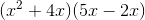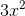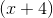Explanation:

This is essentially a multi-part question that at first may seem confusing, until it's realized that the question only involves basic algebra, or more specifically, using FOIL and greatest common factor concepts.

First, we must use FOIL (first, outside, inside, last), to evaluate the given expression: ⋅First: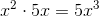Outside: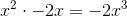Inside: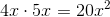Last: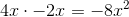Now add all of the terms together: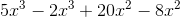Which simplifies to: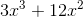Now, we must see what is greatest common factor shared between each of these two terms. They are both divisible byas well as.

Therefore,is the greatest common factor.

### Example Question #6 : How To Use Foil With The Distributive Property

Given that i= –1, what is the value of (6 + 3i)(6 – 3i)?

36 + 9(i2)

36 – 9i

45

36 + 9i

25

45

Explanation:

We start by foiling out the original equation, giving us 36 – 9(i2). Next, substitute 36 – 9(–1). This equals 36 + 9 = 45.

### Example Question #7 : How To Use Foil With The Distributive Property

Expand the following expression:

(B – 2) (B + 4)

B2 + 2B + 8

B2 + 4B – 8

B2 – 2B – 8

B2 – 4B – 8

B2 + 2B – 8

B2 + 2B – 8

Explanation:

Here we use FOIL:

Firsts: B * B = B2

Outer: B * 4 = 4B

Inner: –2 * B = –2B

Lasts: –2 * 4 = –8

All together this yields

B2 + 2B  8

### Example Question #8 : How To Use Foil With The Distributive Property

A rectangle's length L is 3 inches shorter than its width, W. What is an appropriate expression for the area of the rectangle in terms of W?

2W2 – 3

W2 – 3W

W2 – 3

2W – 3

2W2 – 3W

W2 – 3W

Explanation:

The length is equal to W – 3

The area of a rectangle is length x width.

So W * (W – 3) = W2 – 3W

### Example Question #9 : How To Use Foil With The Distributive Property

Expand the following expression:

(f + 4) (f – 4)

f2 – 4f – 16

f2 + 16

2f – 4f – 16

f2 – 16

f2 + 4f – 16

f2 – 16

Explanation:

using FOIL:

First: f x f = f2

Outer: f x – 4 = –4f

Intter: 4 x f = 4f

Lasts: 4 x – 4 = –16

f2 – 4f +4f – 16

### Example Question #10 : How To Use Foil With The Distributive Property

Expand the following expression: (x+3) (x+2)

2x + 5x + 6

x2 + 4x + 6

x2 + 5x + 3

x2 + 5x + 6

x2 + 3x + 6

x2 + 5x + 6

Explanation:

This simply requires us to recall our rules from FOIL

First: X multiplied by X yields x2

Outer: X multplied by 2 yields 2x

Inner: 3 multiplied by x yields 3x

Lasts: 2 multiplied by 3 yields 6

Add it all together and we have x2 + 2x + 3x + 6

← Previous 1 3 4 5 6